# C# Increment | C# Decrement | C# Increment Decrement Operators - c# - c# tutorial - c# net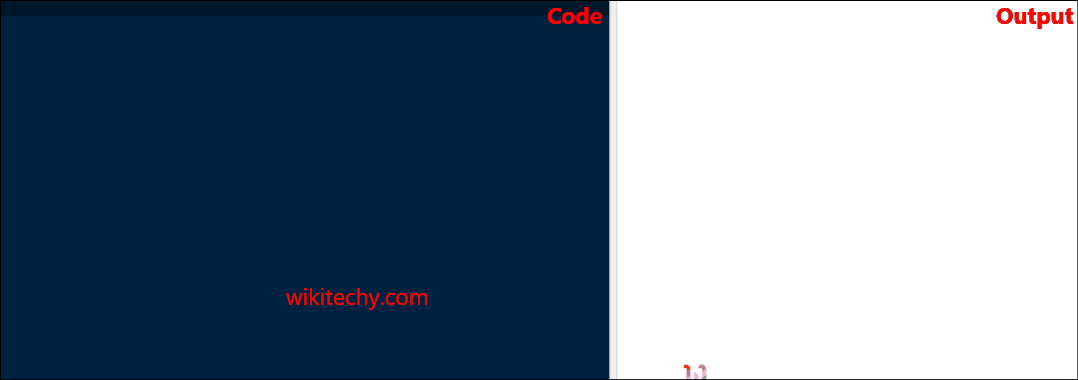C# Increment

## What is the use of Increment and Decrement operator in C# ?

• In C#- Programming Increment operators (++) is used to increase the value of the variable by one.
•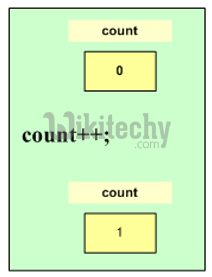• In C#- Programming decrement operators (--) is used to decrease the value of the variable by one.Increment and Decrement Operator

## Syntax: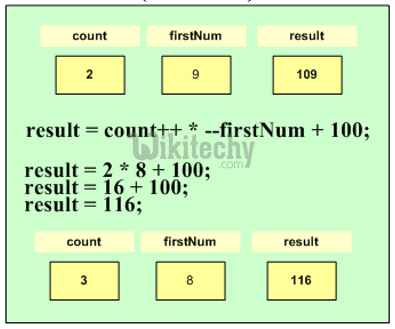## C# Sample Code - C# Examples:

.net internship , best dotnet training , dotnet courses

## Code Explanation: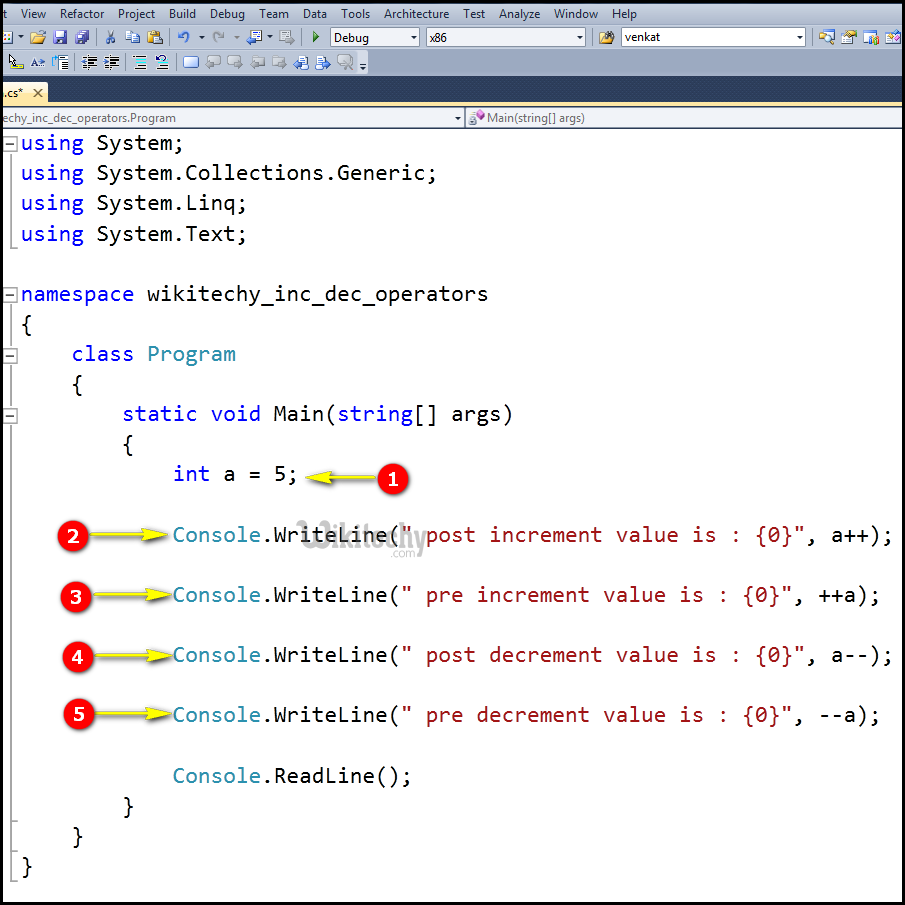1. In this statement we declared and assigned the value "5" for the variable "a".
2. In this statement, the post increment operation will be performed where the "a" value is incremented and stored in the variable(a=5), since it is post increment so the value will be printed only for the current value of "a" (a=5).
3. In this statement, the pre increment operation will be performed where the value of "a=5" because previously the value will be increment which will be stored in the variable "a", so now the value printed in the console display window will be "7" (a=7becasuse of these pre increment).
4. In this statement, the pre decrement operation will be performed where the value of "a" is 7 (a=2 it has been pre decremented), so the value printed in the console display window for "a" will be "7".
5. In this, the printf statement post decrement operation will be performed where the value of "a" is 7, since it is the post decrement operation the value of a=2 is decremented and stored in the variable. In the output the current value of "a=5" will be printed.

## Sample C# examples - Output :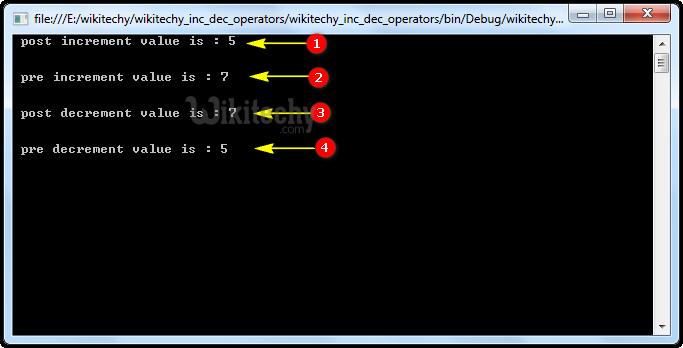1. Here in this output the post increment operation will be performed so the variable "a" value (5) will be displayed.
2. Here in this output the pre increment operation will be performed so the variable "a" value (7) will be displayed.
3. Here in this output the pre decrement operation will be performed so the variable "a" value (7) will be displayed.
4. Here in this output the post decrement operation will be performed so the variable "a" value (5) will be displayed.